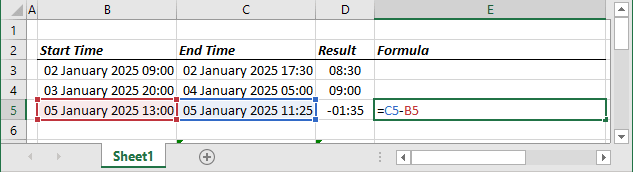# How to format negative timestamps

Excel 365
Excel supports different formats for positive and negative numbers. You can combine text and formatted values to make the display more presentative. Another numeric format is a timestamp, and you can find a difference between two timestamps and display it in days, hours, and minutes. However, Excel does not handle well negative timestamp differences.

All dates and times in Excel are stored as serial numbers, which are nothing but actual numbers. Depending on the system, the date and time can be stored in Excel in two serial number systems:

• The 1900 base system starts from January 1, 1900, as day number 1. This system doesn't allow to work with negative time format. You need to create additional functions to display negative times.
• The 1904 base system (also called Macintosh dates and times) starts from January 1, 1904, as a day number 0. This system works with negative times like regular numbers. See below how to switch to the 1904 date system.

## The 1900 date system

Excel displays correctly only positive time but can’t display negative time: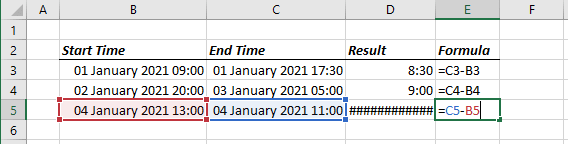To display positive and negative time in the cell, you need to calculate the correct time and add the minus before the negative value:

Type the formula:

= IF (<time> > 0, TEXT (<time>, "hh:mm"), "-" & TEXT (ABS (<time>), "hh:mm"))

where:

• The IF function runs <logical test> and returns one value for a TRUE result, and another for a FALSE result: IF (<logical test>, [<value if true>], [<value if false>]).
• <time> is a result of time calculation.
• "hh:mm" is the format for time.
• & is an operation for concatenating two strings.
• The TEXT function returns <value> to a given <format text>, as text: TEXT (<value>, <format text>).
• The ABS function returns the absolute value of a number (removes the minus sign (-) from a negative number): ABS (<value>).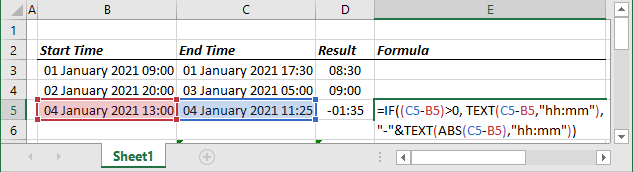## The 1904 date system

When the system changes, all dates in your workbook will be automatically changed: in fact, to every date will be added the difference between January 1, 1900, and January 2, 1904 – 1462 days. All data containing formulas will be changed, as well as charts and diagrams if they use dates!

To change the date system, follow these steps:

1.   On the File tab, click the Options button: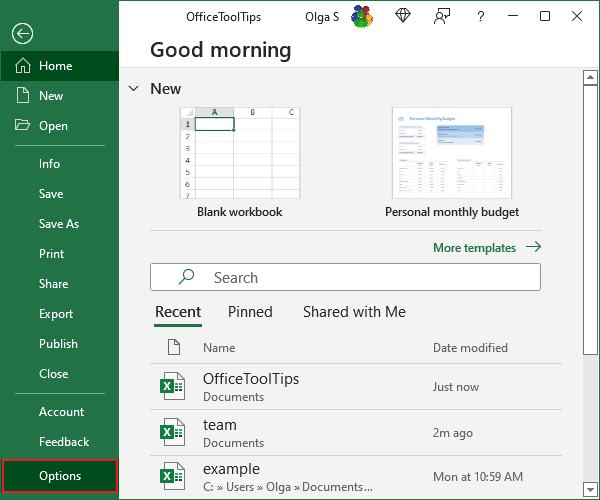2.   In the Excel Options dialog box, in the Advanced tab, under When calculating this workbook, select the Use 1904 date system option: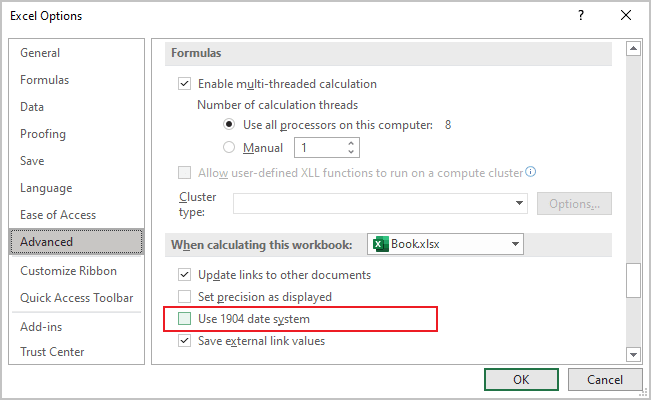Excel works perfectly with negative times in the 1904 date system: